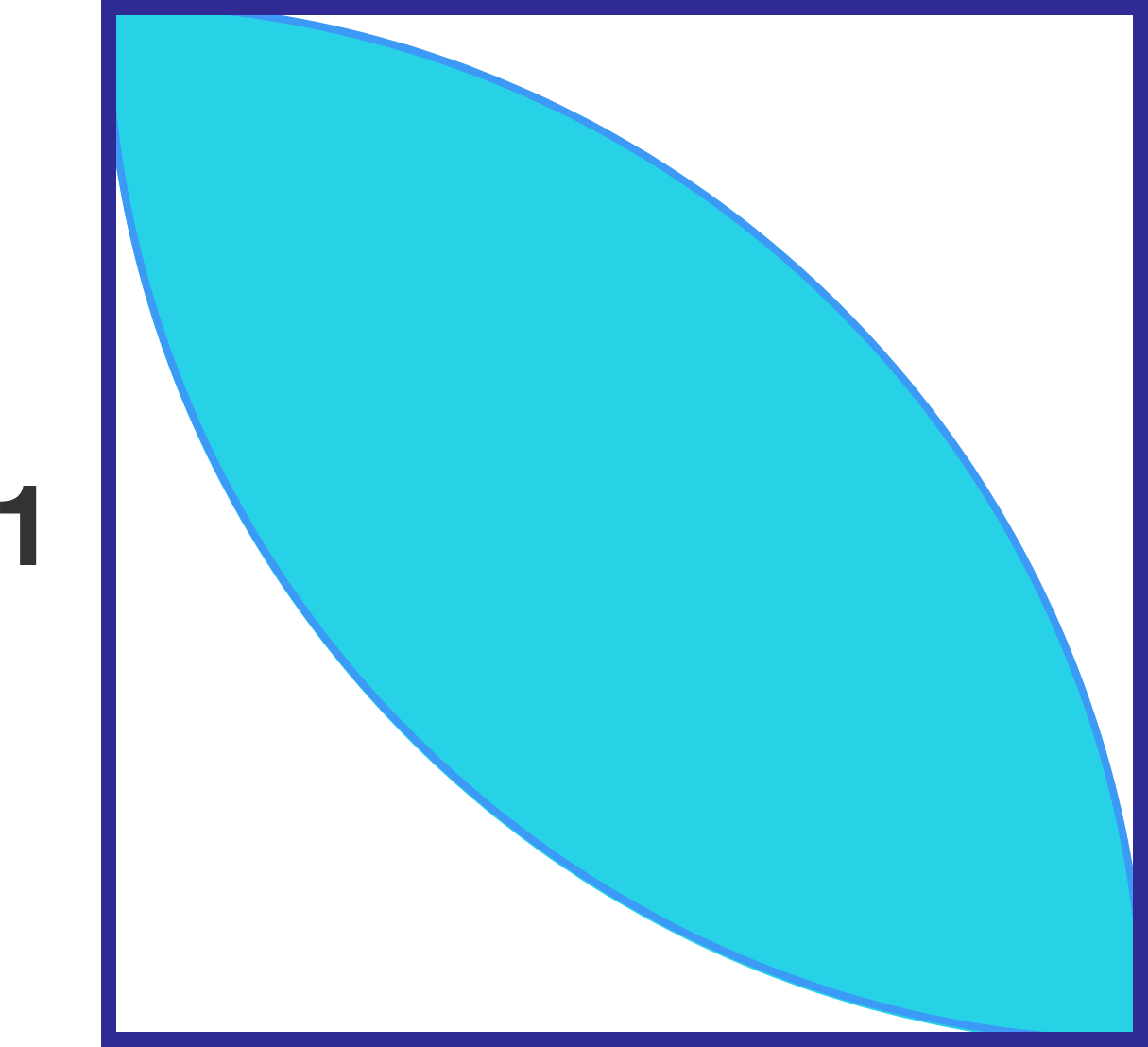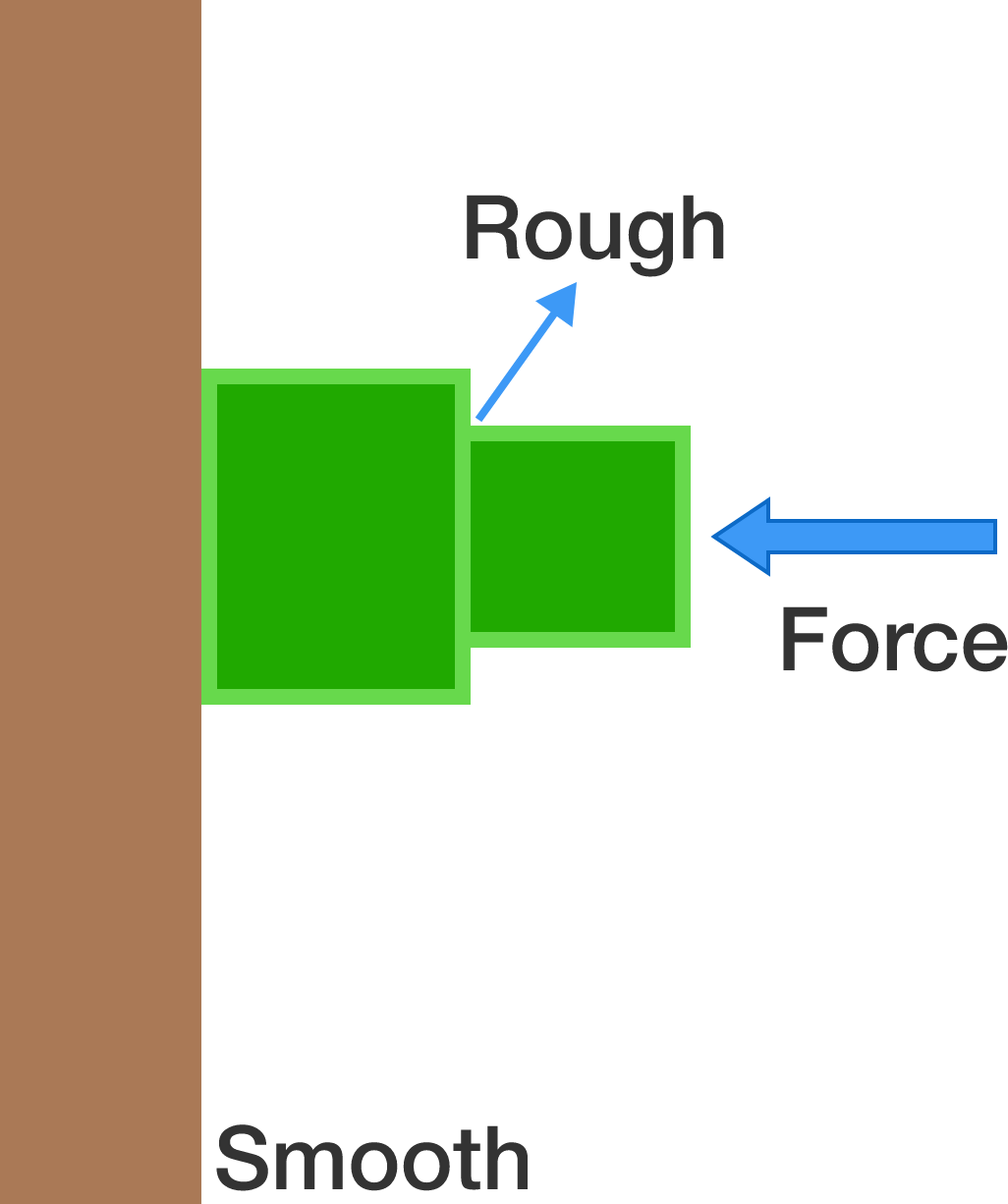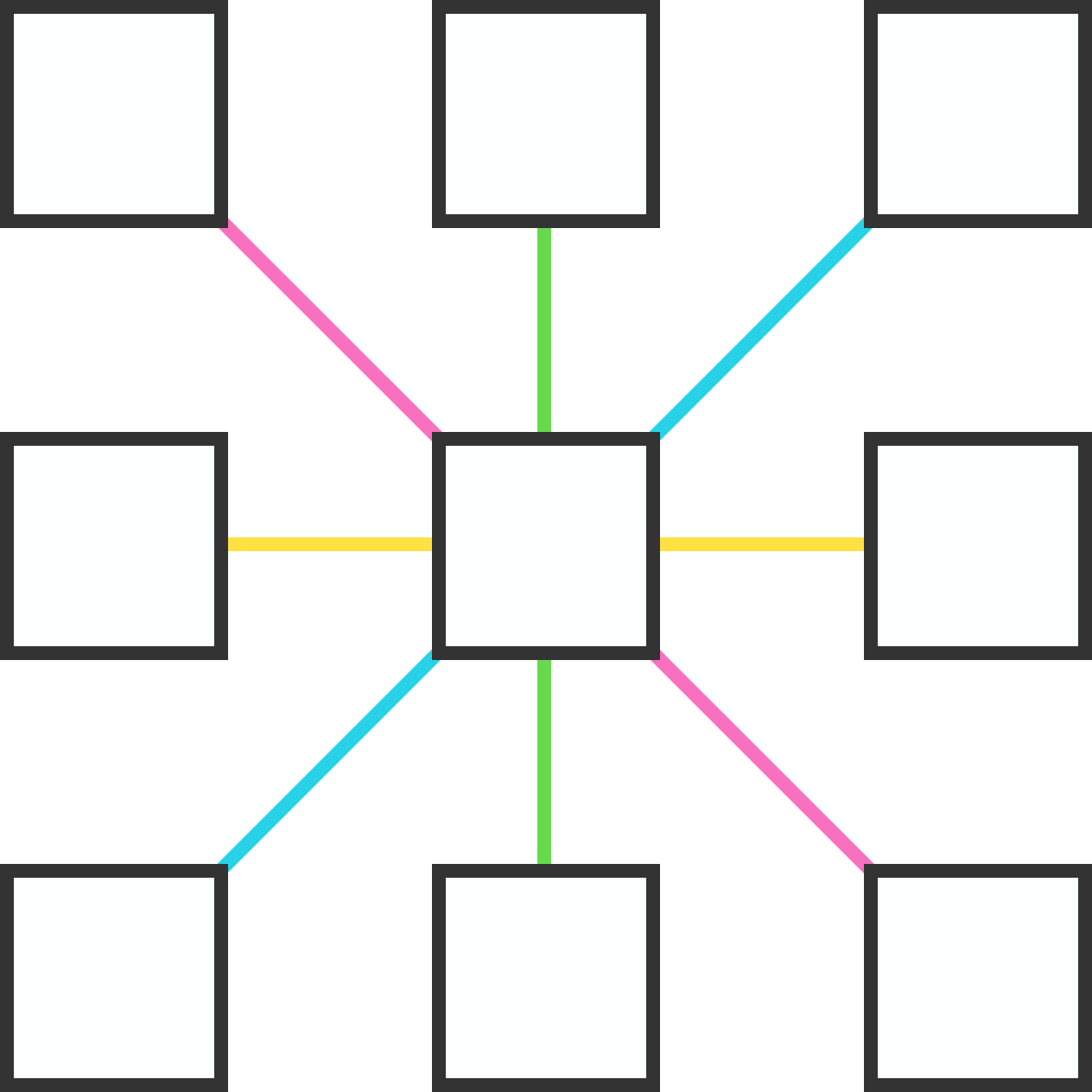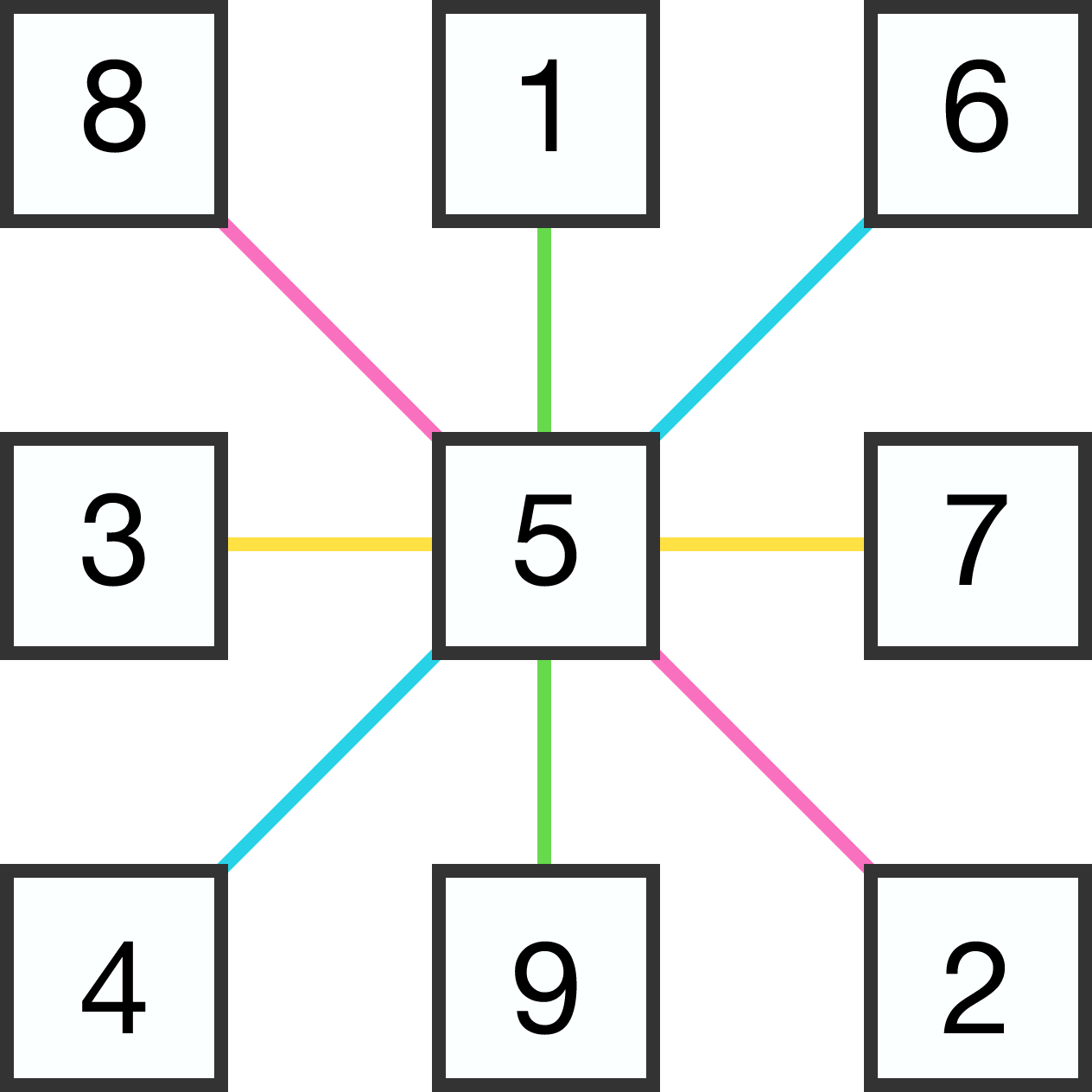# Problems of the Week

Contribute a problem

# 2017-04-31 Basic

Which is larger, $\large \dfrac{ { \color{#3D99F6} 1} } { {\color{#20A900}\frac{2}{3}} }\ \text{ or }\ \dfrac{ {\color{#3D99F6}\frac{ 1 } { 2 }} } { {\color{#20A900}3}}\, ?$In a unit square, two quarter circles are drawn, centered at two opposite vertices.

What is the area of the oval-shaped region?

I will attempt to prove that $\frac00 = 1$. In which of these steps did I first make a mistake by using flawed logic?

Step 1: We can rewrite 15 as $7 + 8$ or $8 + 7$.
Step 2: This means that $7 + 8 = 8+ 7$.
Step 3: If we move one term from each side of the equation to the other side, we will get $7-7 = 8-8.$
Step 4: Dividing both sides by $8-8$ gives $\frac{7-7}{8-8} = 1$.
Step 5: Since $7-7= 0$ and $8-8 = 0$, $\frac00 = 1$.Two blocks are held against a vertical wall by applying a horizontal force, as shown in the diagram. The inner faces of the blocks (where the two blocks make contact) are rough, while all the other surfaces are frictionless. The gravity, of course, acts vertically downwards,

Is it possible for these blocks to remain in equilibrium?Place distinct digits from 1 to 9 in the boxes so that the sums of 3 digits along any of the 4 colored lines are all equal.

What is the maximum possible sum that can be achieved?

For example, using the values in the magic square gives us a sum of 15 along each colored line. Can we do better?×